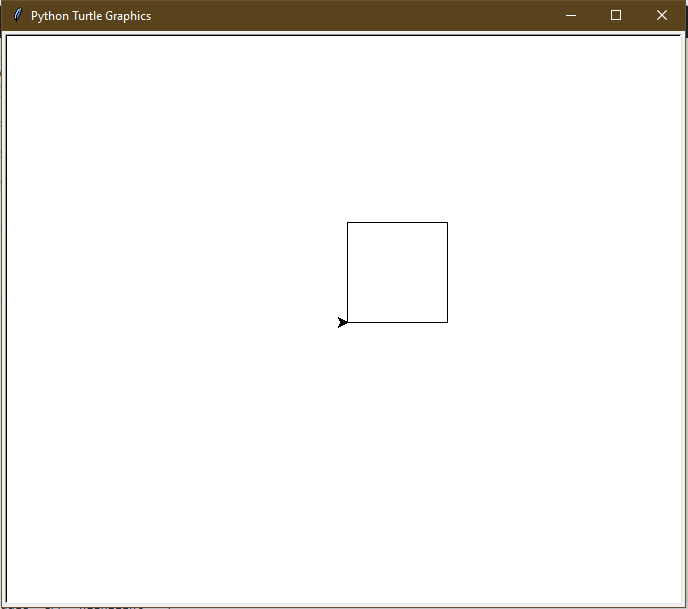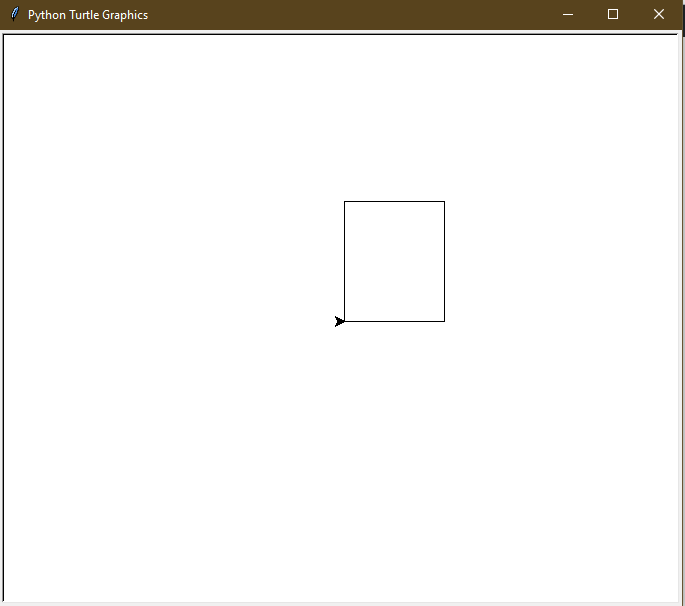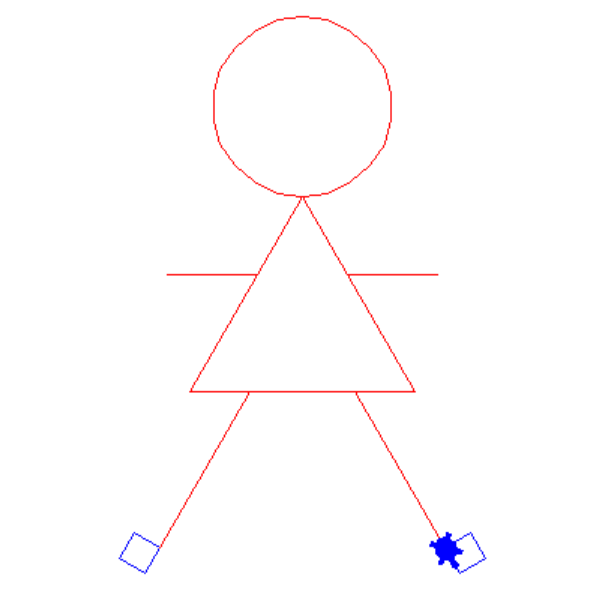# Draw Square and Rectangle in Turtle – Python

Prerequisite: Turtle Programming Basics
turtle is an inbuilt module in Python. It provides drawing using a screen (cardboard) and turtle (pen). To draw something on the screen, we need to move the turtle (pen). To move turtle, there are some functions i.e forward(), backward(), etc.

## Python3

 `# draw square in Python Turtle` `import` `turtle` ` `  `t ``=` `turtle.Turtle()`   `s ``=` `int``(``input``(``"Enter the length of the side of the Squre: "``))`   `# drawing first side` `t.forward(s) ``# Forward turtle by s units` `t.left(``90``) ``# Turn turtle by 90 degree`   `# drawing second side` `t.forward(s) ``# Forward turtle by s units` `t.left(``90``) ``# Turn turtle by 90 degree`   `# drawing third side` `t.forward(s) ``# Forward turtle by s units` `t.left(``90``) ``# Turn turtle by 90 degree`   `# drawing fourth side` `t.forward(s) ``# Forward turtle by s units` `t.left(``90``) ``# Turn turtle by 90 degree`

Input :

```100

```

Output :Second approach (Using Loop) :

## Python3

 `# draw Squre in Python Turtle` `import` `turtle` ` `  `t ``=` `turtle.Turtle()`   `s ``=` `int``(``input``(``"Enter the length of the side of squre: "``))`   `for` `_ ``in` `range``(``4``):` `  ``t.forward(s) ``# Forward turtle by s units` `  ``t.left(``90``) ``# Turn turtle by 90 degree`

Input :

```100

```

Output :## Python3

 `# draw Rectangle in Python Turtle` `import` `turtle` ` `  `t ``=` `turtle.Turtle()`   `l ``=` `int``(``input``(``"Enter the length of the Rectangle: "``))` `w ``=` `int``(``input``(``"Enter the width of the Rectangle: "``))`   `# drawing first side` `t.forward(l) ``# Forward turtle by l units` `t.left(``90``) ``# Turn turtle by 90 degree`   `# drawing second side` `t.forward(w) ``# Forward turtle by w units` `t.left(``90``) ``# Turn turtle by 90 degree`   `# drawing third side` `t.forward(l) ``# Forward turtle by l units` `t.left(``90``) ``# Turn turtle by 90 degree`   `# drawing fourth side` `t.forward(w) ``# Forward turtle by w units` `t.left(``90``) ``# Turn turtle by 90 degree`

Input :

```100
120

```

Output :Second approach (Using Loop) :

## Python3

 `# draw Rectangle in Python Turtle` `import` `turtle` ` `  `t ``=` `turtle.Turtle()`   `l ``=` `int``(``input``(``"Enter the length of the Rectangle: "``))` `w ``=` `int``(``input``(``"Enter the width of the Rectangle: "``))`   `for` `_ ``in` `range``(``4``):` `  `  `  ``# drawing length` `  ``if` `_``%` `2` `=``=` `0``:` `    ``t.forward(l) ``# Forward turtle by l units` `    ``t.left(``90``) ``# Turn turtle by 90 degree`   `  ``# drawing width` `  ``else``:` `    ``t.forward(w) ``# Forward turtle by w units` `    ``t.left(``90``) ``# Turn turtle by 90 degree`

Input :

```100
120

```

Output :Since as of now, you must have learned how to draw various basic geometrical illustrations like circle, square, rectangle. So, let’s implement this knowledge to build something which you can really use in building games like let’s draw a human being using the basic knowledge of geometrical knowledge.

Here the code for this implementation:-

## Python3

 `import` `turtle`   `def` `draw_dream():` `    ``window ``=` `turtle.Screen()` `    ``window.bgcolor(``"white"``)` `    ``draw_Scarlett()` `    ``window.exitonclick()`     `def` `draw_Scarlett():` `    ``brad ``=` `turtle.Turtle()` `    ``brad.shape(``"turtle"``)` `    ``brad.color(``"red"``)` `    ``draw_head(brad)` `    ``draw_body(brad)` `    ``draw_arm(brad)` `    ``draw_leg1(brad)` `    ``draw_leg2(brad)`     `def` `draw_head(brad):` `    ``brad.circle(``60``)` `    ``brad.speed(``3``)` `    ``brad.right(``60``)`     `def` `draw_body(brad):` `    ``num ``=` `0` `    ``while` `num < ``3``:` `        ``brad.forward(``150``)` `        ``brad.right(``120``)` `        ``brad.speed(``1``)` `        ``num ``+``=` `1`     `def` `draw_arm(brad):` `    ``brad.forward(``60``)` `    ``brad.left(``60``)` `    ``brad.forward(``60``)`   `    ``brad.backward(``60``)` `    ``brad.right(``60``)` `    ``brad.backward(``60``)`   `    ``brad.right(``60``)`   `    ``brad.forward(``60``)` `    ``brad.right(``60``)` `    ``brad.forward(``60``)`   `    ``brad.backward(``60``)` `    ``brad.left(``60``)` `    ``brad.forward(``90``)`     `def` `draw_leg1(brad):` `    ``brad.left(``120``)` `    ``brad.forward(``40``)` `    ``brad.right(``120``)` `    ``brad.forward(``120``)` `    ``draw_foot1(brad)`     `def` `draw_leg2(brad):` `    ``brad.color(``"red"``)` `    ``brad.right(``180``)` `    ``brad.forward(``120``)` `    ``brad.right(``60``)` `    ``brad.forward(``70``)` `    ``brad.right(``60``)` `    ``brad.forward(``120``)` `    ``draw_foot2(brad)`     `def` `draw_foot1(brad):` `    ``brad.color(``"blue"``)` `    ``num ``=` `0` `    ``while` `num < ``4``:` `        ``brad.forward(``20``)` `        ``brad.right(``90``)` `        ``num ``+``=` `1`     `def` `draw_foot2(brad):` `    ``brad.color(``"blue"``)` `    ``num ``=` `0` `    ``while` `num < ``4``:` `        ``brad.forward(``20``)` `        ``brad.left(``90``)` `        ``num ``+``=` `1`     `draw_dream()`

Output:My Personal Notes arrow_drop_upCheck out this Author's contributed articles.

If you like GeeksforGeeks and would like to contribute, you can also write an article using contribute.geeksforgeeks.org or mail your article to contribute@geeksforgeeks.org. See your article appearing on the GeeksforGeeks main page and help other Geeks.

Please Improve this article if you find anything incorrect by clicking on the "Improve Article" button below.

Improved By : anshitaagarwal

Article Tags :

2

Please write to us at contribute@geeksforgeeks.org to report any issue with the above content.